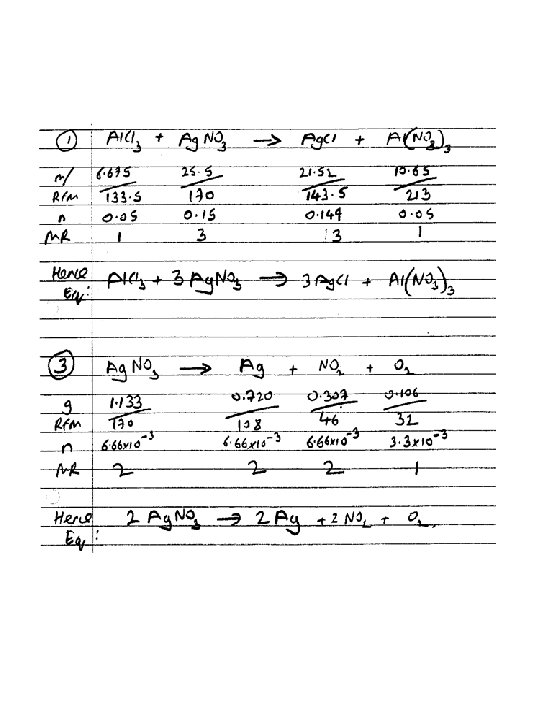# Calculations in Chemistry You need to know how

• Slides: 14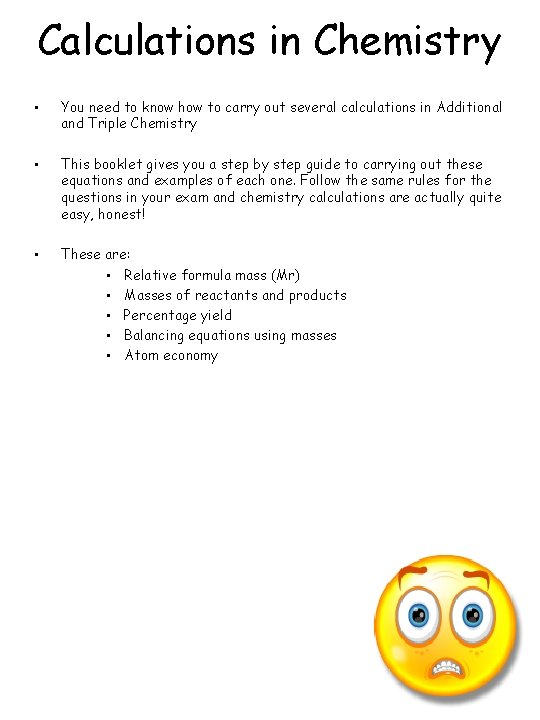Calculations in Chemistry • You need to know how to carry out several calculations in Additional and Triple Chemistry • This booklet gives you a step by step guide to carrying out these equations and examples of each one. Follow the same rules for the questions in your exam and chemistry calculations are actually quite easy, honest! • These are: • Relative formula mass (Mr) • Masses of reactants and products • Percentage yield • Balancing equations using masses • Atom economy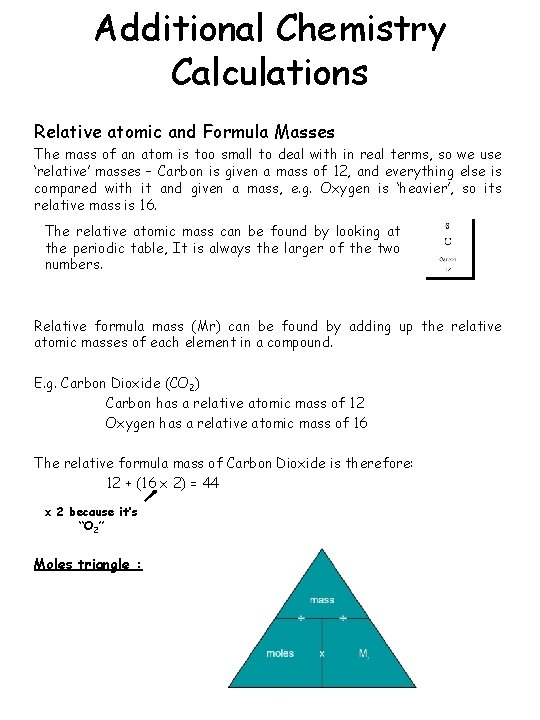Additional Chemistry Calculations Relative atomic and Formula Masses The mass of an atom is too small to deal with in real terms, so we use ‘relative’ masses – Carbon is given a mass of 12, and everything else is compared with it and given a mass, e. g. Oxygen is ‘heavier’, so its relative mass is 16. The relative atomic mass can be found by looking at the periodic table, It is always the larger of the two numbers. Relative formula mass (Mr) can be found by adding up the relative atomic masses of each element in a compound. E. g. Carbon Dioxide (CO 2) Carbon has a relative atomic mass of 12 Oxygen has a relative atomic mass of 16 The relative formula mass of Carbon Dioxide is therefore: 12 + (16 x 2) = 44 x 2 because it’s “O 2” Moles triangle :Find the Mr of: 1. Na. Cl 2. Pb (OH)2 3. Mg. SO 4 4. g(NO 3)2 Example exam question: Calculate the relative formula mass (Mr) of carbon monoxide. Relative atomic masses (Ar): C = 12; O = 16 Mr of carbon monoxide =. . . . (1)Masses of reactants and products This is an important calculation when we want to know how much of each reactant to react together. For example, sodium hydroxide reacts with chlorine gas to make bleach. If we have too much Chlorine, some will be wasted. Too little and not all of the sodium hydroxide will react. 2 Na. OH + Cl 2 Na. OCl + Na. Cl + H 2 O Sodium Chlorine Bleach Hydroxide Salt Water How much Chlorine gas should we bubble through 100 g of Sodium Hydroxide to make Bleach? Cl 2 Na. OCl + Na. Cl + H 2 O 2 Na. OH + 100 g Work out Mr: 23 +16+1 =40 Mass/mr = moles 100/40 = 2. 5 ? 35. 5 x 2 =70 Look at ratio: 2 : Scale moles into ratio 2. 5 moles : Multiply moles by Mr 1 1. 25 moles = 1. 25 x 70 = 87. 5 g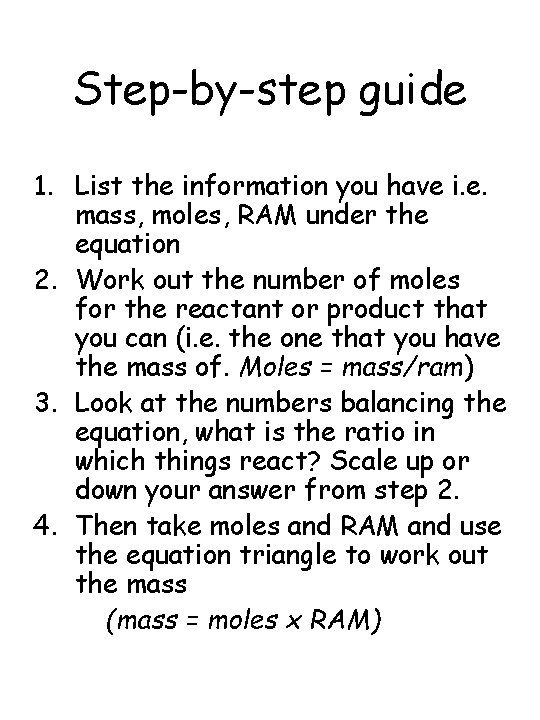Step-by-step guide 1. List the information you have i. e. mass, moles, RAM under the equation 2. Work out the number of moles for the reactant or product that you can (i. e. the one that you have the mass of. Moles = mass/ram) 3. Look at the numbers balancing the equation, what is the ratio in which things react? Scale up or down your answer from step 2. 4. Then take moles and RAM and use the equation triangle to work out the mass (mass = moles x RAM)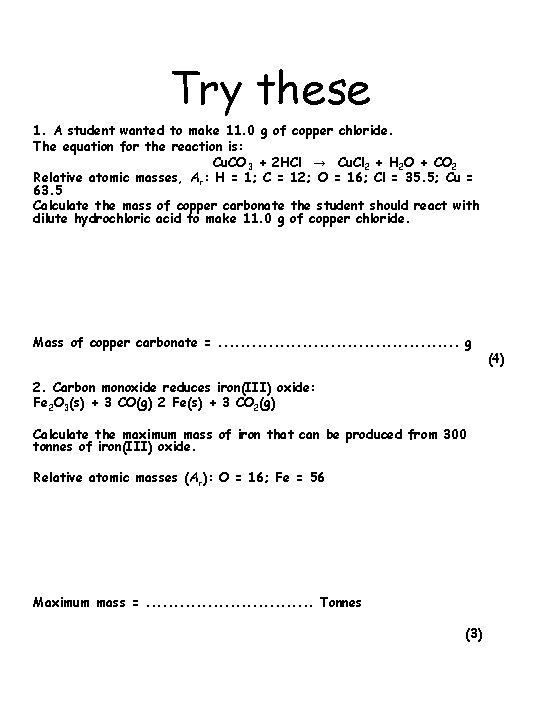Try these 1. A student wanted to make 11. 0 g of copper chloride. The equation for the reaction is: Cu. CO 3 + 2 HCl → Cu. Cl 2 + H 2 O + CO 2 Relative atomic masses, Ar: H = 1; C = 12; O = 16; Cl = 35. 5; Cu = 63. 5 Calculate the mass of copper carbonate the student should react with dilute hydrochloric acid to make 11. 0 g of copper chloride. Mass of copper carbonate =. . . g 2. Carbon monoxide reduces iron(III) oxide: Fe 2 O 3(s) + 3 CO(g) 2 Fe(s) + 3 CO 2(g) Calculate the maximum mass of iron that can be produced from 300 tonnes of iron(III) oxide. Relative atomic masses (Ar): O = 16; Fe = 56 Maximum mass =. . . . Tonnes (3) (4)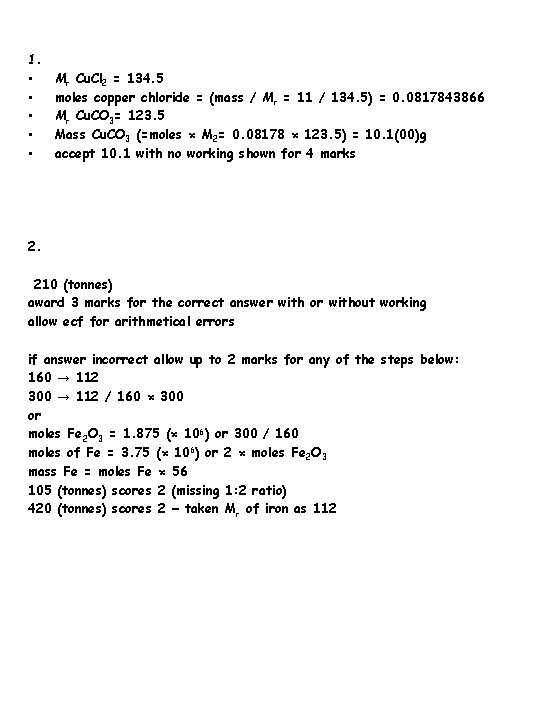1. • • • Mr Cu. Cl 2 = 134. 5 moles copper chloride = (mass / Mr = 11 / 134. 5) = 0. 0817843866 Mr Cu. CO 3= 123. 5 Mass Cu. CO 3 (=moles × M 2= 0. 08178 × 123. 5) = 10. 1(00)g accept 10. 1 with no working shown for 4 marks 2. 210 (tonnes) award 3 marks for the correct answer with or without working allow ecf for arithmetical errors if answer incorrect allow up to 2 marks for any of the steps below: 160 → 112 300 → 112 / 160 × 300 or moles Fe 2 O 3 = 1. 875 (× 106) or 300 / 160 moles of Fe = 3. 75 (× 106) or 2 × moles Fe 2 O 3 mass Fe = moles Fe × 56 105 (tonnes) scores 2 (missing 1: 2 ratio) 420 (tonnes) scores 2 − taken Mr of iron as 112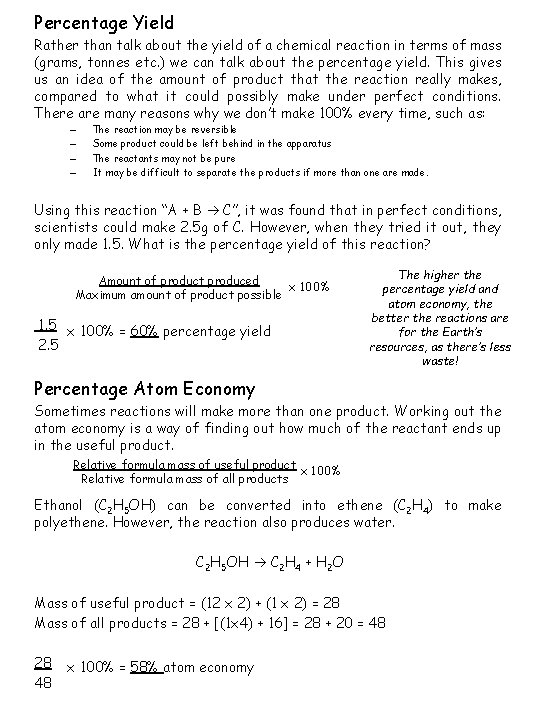Percentage Yield Rather than talk about the yield of a chemical reaction in terms of mass (grams, tonnes etc. ) we can talk about the percentage yield. This gives us an idea of the amount of product that the reaction really makes, compared to what it could possibly make under perfect conditions. There are many reasons why we don’t make 100% every time, such as: – – The reaction may be reversible Some product could be left behind in the apparatus The reactants may not be pure It may be difficult to separate the products if more than one are made. Using this reaction “A + B C”, it was found that in perfect conditions, scientists could make 2. 5 g of C. However, when they tried it out, they only made 1. 5. What is the percentage yield of this reaction? Amount of product produced x 100% Maximum amount of product possible 1. 5 x 100% = 60% percentage yield 2. 5 The higher the percentage yield and atom economy, the better the reactions are for the Earth’s resources, as there’s less waste! Percentage Atom Economy Sometimes reactions will make more than one product. Working out the atom economy is a way of finding out how much of the reactant ends up in the useful product. Relative formula mass of useful product x 100% Relative formula mass of all products Ethanol (C 2 H 5 OH) can be converted into ethene (C 2 H 4) to make polyethene. However, the reaction also produces water. C 2 H 5 OH C 2 H 4 + H 2 O Mass of useful product = (12 x 2) + (1 x 2) = 28 Mass of all products = 28 + [(1 x 4) + 16] = 28 + 20 = 48 28 48 x 100% = 58% atom economy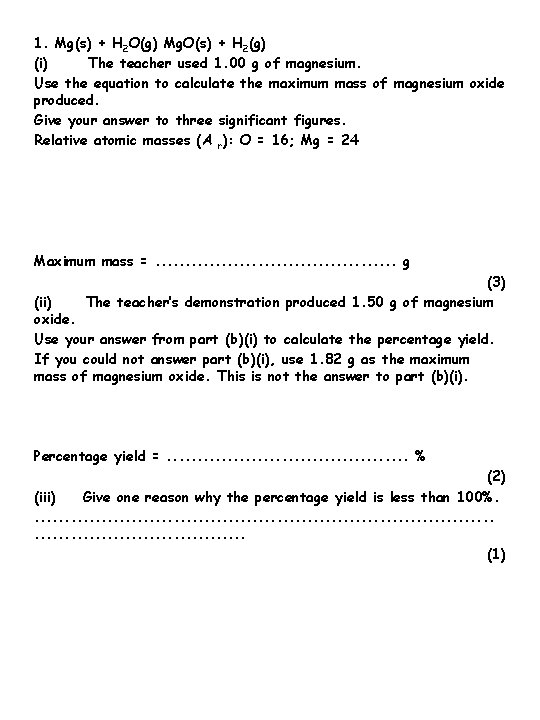1. Mg(s) + H 2 O(g) Mg. O(s) + H 2(g) (i) The teacher used 1. 00 g of magnesium. Use the equation to calculate the maximum mass of magnesium oxide produced. Give your answer to three significant figures. Relative atomic masses (A r): O = 16; Mg = 24 Maximum mass =. . . . . g (3) (ii) The teacher’s demonstration produced 1. 50 g of magnesium oxide. Use your answer from part (b)(i) to calculate the percentage yield. If you could not answer part (b)(i), use 1. 82 g as the maximum mass of magnesium oxide. This is not the answer to part (b)(i). Percentage yield =. . . . . % (2) (iii) Give one reason why the percentage yield is less than 100%. . . . . . . (1)1. 67(g) allow 1. 66 -1. 68 correct answer (to 3 significant figures) with or without working gains 3 marks if answer incorrect allow up to 2 marks for the following steps: 24 → 40 1. 00 → 40 / 24 or moles magnesium = 1 / 24 or 0. 04(17) multiply by 40 allow ecf from incorrect ratio or incorrect number of moles 3 (ii) if correct answer from part (b)(i) used allow ecf from part (b)(i) 89. 8 or 90 if 1. 82 g used 82. 4 or 82 correct answer with or without working gains 2 marks if answer incorrect, allow the following for 1 mark: 1. 50 / 1. 67 (or their answer from part (b)(i)) if 1. 82 g used: 1. 50 / 1. 82 (iii) any one from: ignore measurement errors • not all the magnesium reacted allow the reaction may be reversible • some of the magnesium oxide / product may have been left in the tube or may have been lost ignore magnesium lost • different / unexpected reaction • magnesium not pure 1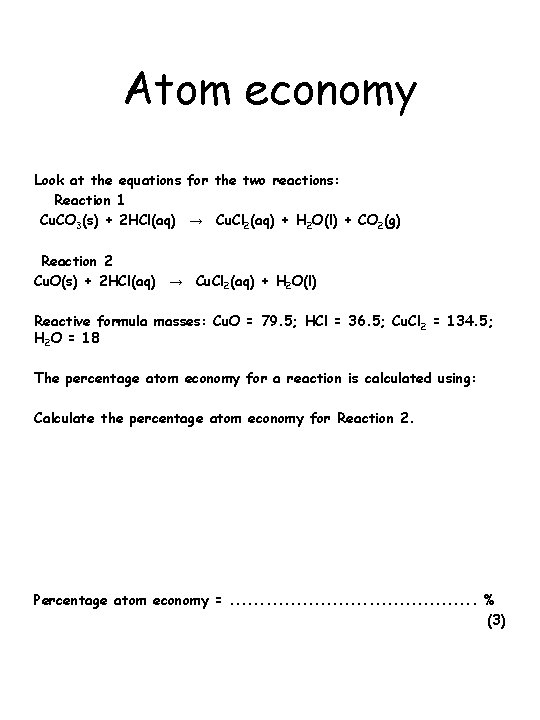Atom economy Look at the equations for the two reactions: Reaction 1 Cu. CO 3(s) + 2 HCl(aq) → Cu. Cl 2(aq) + H 2 O(l) + CO 2(g) Reaction 2 Cu. O(s) + 2 HCl(aq) → Cu. Cl 2(aq) + H 2 O(l) Reactive formula masses: Cu. O = 79. 5; HCl = 36. 5; Cu. Cl 2 = 134. 5; H 2 O = 18 The percentage atom economy for a reaction is calculated using: Calculate the percentage atom economy for Reaction 2. Percentage atom economy =. . . . . % (3)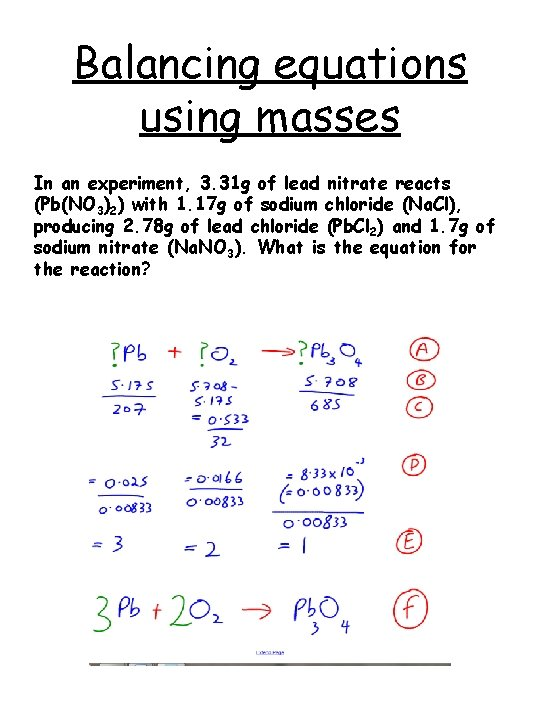Balancing equations using masses In an experiment, 3. 31 g of lead nitrate reacts (Pb(NO 3)2) with 1. 17 g of sodium chloride (Na. Cl), producing 2. 78 g of lead chloride (Pb. Cl 2) and 1. 7 g of sodium nitrate (Na. NO 3). What is the equation for the reaction?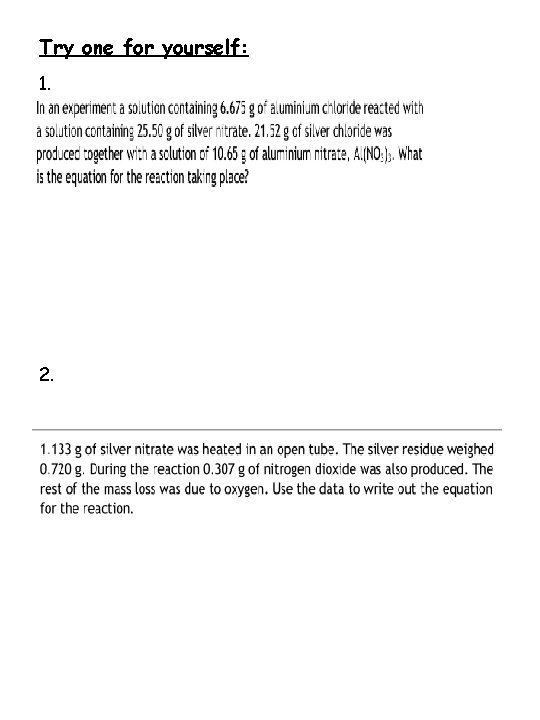Try one for yourself: 1. 2.